## Sunday, March 8, 2009

### QTP Reading from Datatable

Reading a value from an action's own datatable

Msgbox DataTable.Value("A", dtLocalSheet)
or
Msgbox DataTable.Value("A", 2)
or
Msgbox DataTable.Value("A", "Action1")Reading a value from Global datatable

Msgbox DataTable.Value("A", dtGlobalSheet)
or
Msgbox DataTable.Value("A", 1)
or
Msgbox DataTable.Value("A", Global)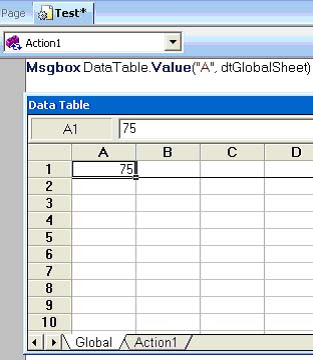Another way of reading a value from a datatable from Global Sheet
[Column name is A and Sheet id is 1, i.e. Global Sheet]
Msgbox Datatable ("A" , 1 )
or
Msgbox Datatable("A", dtGlobalSheet)
or
Msgbox Datatable("A", Global)Another way of reading a value from a datatable from Local Sheet
[Column name is A and Sheet id is 2, i.e. Local Sheet]
Msgbox Datatable ("A" , 2 )
or
Msgbox Datatable ("A" , "Action1")
or
Msgbox Datatable ("A", dtLocalSheet)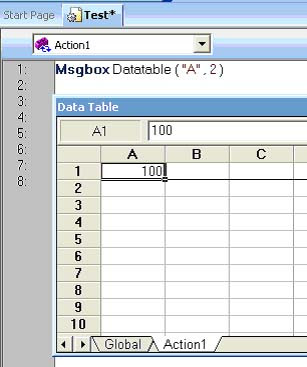Reading a value from another action's datatable

Msgbox DataTable.GetSheet("Action2").GetParameter("A").Value
[See the below screenshot – code is written in Action1 and this code will show value in Action2's Sheet i.e. 11]Msgbox DataTable.GetSheet("Global").GetParameter("A").Value
[See the below screenshot – code is written in Action1 and this code will show value in Global Sheet i.e. 55]Msgbox DataTable.GetSheet(1).GetParameter("A").Value
[See the below screenshot – code is written in Action1 and this code will show value in Global Sheet i.e. 55]Reading a value from external action's datatable

Msgbox DataTable.GetSheet("sac [rd]").GetParameter("A").Value
[See the last screenshot – code is written in Action1 and this code will show value from Sheet of External Action i.e. 14]

A new test which has only one action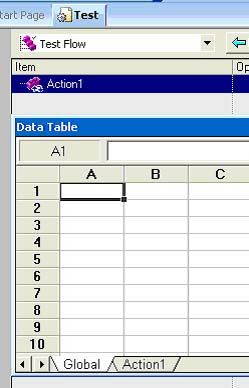Calling an external action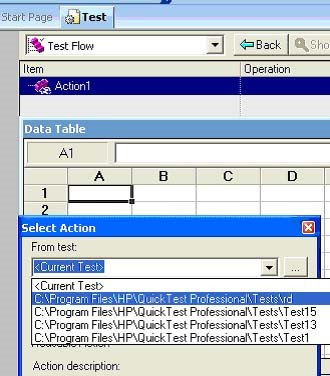Now external action named “sac” (from test “rd”) is added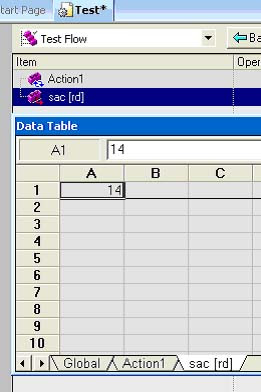Retrieving a value of the cell from the specified row of the datatable

Msgbox (DataTable.GetSheet(2).GetParameter("A").ValueByRow(3))
[
It will show 81]
or
Msgbox (DataTable.GetSheet("Action1").GetParameter("A").ValueByRow(3))
or
Msgbox (DataTable.GetSheet("Global").GetParameter("A").ValueByRow(3))Retrieving a value of the cell in the specified row of the Global or Local datatable

Msgbox(DataTable.GlobalSheet.GetParameter("A").ValueByRow(3))
[
It will show 78]
Or
Msgbox(DataTable.LocalSheet.GetParameter("A").ValueByRow(3))Retrieving a value of the cell in the specified row of the External Sheet
[See the below screenshot – code is written in Action1 and this code will show value in sac [rd] Sheet i.e. 14, “sac” is an Action in test named “rd”]
Msgbox(DataTable.GetSheet(3).GetParameter("A").ValueByRow(1))Also See:

QTP Data Table Basics

Writing Values To Data Table

How to import excel sheet into data table in QTP

Accessing Data Table through scripts

Multiple Choice Questions on Data Table

Table Checkpoints Multiple Choice Questions

Data Table Menu Shortcut Keys